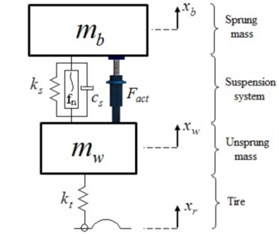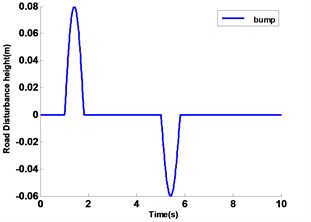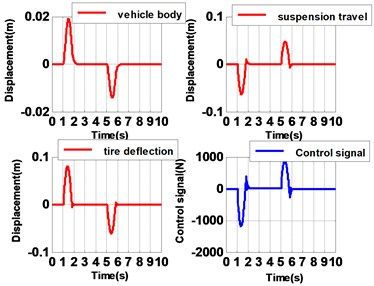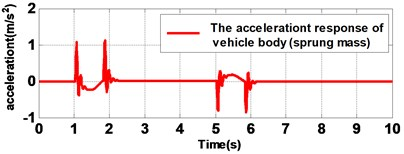Published: 20 April 2018

# Adaptive sliding neural network-based vibration control of a nonlinear quarter car active suspension system with unknown dynamics

Peyman Ghahremani3
2, 3Khajeh Nassir Toosi University of Technology, Tehran, Iran
2, 3University of Minnesota, Minneapolis, USA
Corresponding Author:
Views 177

#### Abstract

This study investigates adaptive sliding neural network (NN) control for quarter active suspension system with dynamic uncertainties and road disturbances. A Multilayer Perceptron (MLP) neural network is adopted to estimate the unknown dynamics of the system. In addition, sliding mode controller is introduced to compensate the function of estimation error for improving the performance of the system. Furthermore, the uniformly and bounded of closed-loop signals is guaranteed by Lyapunov analysis; the adaptation laws for training of MLP are derived from stability analysis. The simulation results demonstrate that the proposed controller can effectively provide a good ride and makes great trade-off between passenger comfort and handling despite the dynamic uncertainties.

## 2. Problem formulation

The proposed nonlinear quarter car suspension system with two degree of freedom dynamic model is shown in Fig. 1, despite the fact that quarter car suspension model is simple, but it is comprehensive to simulate and adopt different characteristics of real suspension system.

Fig. 1A nonlinear quarter car suspension modelThe suspension system is like a bridge between the sprung ${m}_{b}$ and unsprung ${m}_{w}$ masses, ${x}_{b}$ and ${x}_{w}$ are vertical displacements of sprung and unsprung masses; and ${x}_{r}$ is the road profile. The state space equation of motion for this suspension system can be expressed as:

1
${m}_{b}{\stackrel{¨}{x}}_{b}+{c}_{s}\left({\stackrel{˙}{x}}_{b}-{\stackrel{˙}{x}}_{w}\right)+{k}_{s}\left({x}_{b}-{x}_{w}\right)+{f}_{n}={F}_{act},$
2
${m}_{w}{\stackrel{¨}{x}}_{w}-{c}_{s}\left({\stackrel{˙}{x}}_{b}-{\stackrel{˙}{x}}_{w}\right)-{k}_{s}\left({x}_{b}-{x}_{w}\right)+{k}_{t}\left({x}_{w}-{x}_{r}\right)-{f}_{n}={-F}_{act}.$

In this research, we consider the dynamic of the system unknown and our proposed controller cannot be affected by uncertainties. The linear stiffness of suspension spring is defined as ${k}_{s}$ and stiffness of tire denoted as ${k}_{t}$, damping constant of suspension is named ${c}_{s}$. The nonlinear characteristic of suspension is ${f}_{n}$ which can model the cubic stiffness and ${k}_{s}^{n}$ is the nonlinear spring coefficient, the new controller is not sensitive by changing constants and masses and so on; and our new controller can estimate the unknown dynamics of the system:

3
${f}_{n}={k}_{s}^{n}{\left({x}_{b}-{x}_{w}\right)}^{3}.$

The states of the quarter suspension are defined as:

4
$X=\left[\begin{array}{cc}{x}_{b}& {x}_{w}\end{array}\right].$

The nonlinear quarter car model dynamics is defined as follows:

5
$M\stackrel{¨}{x}=F+Gu,$

where:

6
$F=\left[\begin{array}{c}{F}_{1}\\ {F}_{2}\end{array}\right],$
7
${F}_{1}=-{c}_{s}\left({\stackrel{˙}{x}}_{b}-{\stackrel{˙}{x}}_{w}\right)-{k}_{s}\left({x}_{b}-{x}_{w}\right)-{k}_{s}^{n}{\left({x}_{b}-{x}_{w}\right)}^{3},$
8
${F}_{2}={c}_{s}\left({\stackrel{˙}{x}}_{b}-{\stackrel{˙}{x}}_{w}\right)+{k}_{s}\left({x}_{b}-{x}_{w}\right)-{k}_{t}\left({x}_{w}-{x}_{r}\right)+{k}_{s}^{n}{\left({x}_{b}-{x}_{w}\right)}^{3},$
9
$M=\left[\begin{array}{cc}{m}_{b}& 0\\ 0& {m}_{w}\end{array}\right].$

Term $u$ is used for estimation of actuator force which is represented as follows:

10
$u={F}_{act}.$

## 3. Neural network controller

In the control engineering, a Neural Network (NN) is usually utilized to approximate structure or parametric uncertainties , The structure of Multilayer Perception (MLP) has been proposed in the design of this combinational controller, consists of three layers input, hidden and output. Three-layer neural network is employed to approximate the uncertain dynamic in the model of system. The output of this NN can be obtained:

11
${net}_{h}={w}_{h}^{T}{u}_{nn},$
12
${o}_{h}={f}_{h}\left({w}_{h}^{T}{u}_{nn}\right),$
13
$o={w}_{o}^{T}{o}_{h},$
14
${f}_{h}\left(x\right)=\frac{1-\mathrm{e}\mathrm{x}\mathrm{p}\left(-x\right)}{1+\mathrm{e}\mathrm{x}\mathrm{p}\left(-x\right)}.$

Let Taylor series expansion of the function $o$ be:

15
$o={\theta }^{T}\xi ,$

where $\theta$ is weight vector of hidden and output layer, $\xi$ is the derivative of output with respect to $\theta$ parameters.

## 4. Design of sliding mode controller

To evaluate the performance of suspension system with help of NN for estimating the unknown dynamics has been studied. As the first step, a stable sliding surface is defined as:

16
$s=\stackrel{˙}{x}+\lambda x.$

In which $\lambda$ is a positive constant, multiplying both sides of Eq. (16) in matrix $M$:

17
$Ms=M\stackrel{˙}{x}+M\lambda x.$

By differentiating Eq. (17) and replacing that in Eq. (5) results in:

18
$M\stackrel{˙}{s}=F+Gu+M\lambda x.$

Eq. (9) can be rewritten as follows:

19
$M\stackrel{˙}{s}=H+Gu,$
20
$H=F+M\lambda \stackrel{˙}{x}.$

In Eq. (18) has been assumed been assumed $H$ is uncertain and unknown, which can be estimated by NN; on the other hand, $\stackrel{^}{H}={\left[\begin{array}{cc}{\stackrel{^}{H}}_{1}& {\stackrel{^}{H}}_{2}\end{array}\right]}^{T}$ and ${\stackrel{^}{H}}_{1}$, ${\stackrel{^}{H}}_{2}$ are Neural Networks of MLP.

According to Eq. (10) and considering estimation of $H$ by NN, control signal can be represented as follows:

21
$Gu=-ks-\stackrel{^}{H}-\eta \mathrm{s}\mathrm{i}\mathrm{g}\mathrm{n}\left(s\right).$

In which $k$ and $\eta$ are positive parametric vectors. By replacing Eq. (21) in Eq. (19) which yields:

22
$M\stackrel{˙}{s}=H-\stackrel{^}{H}-ks-\eta \mathrm{s}\mathrm{i}\mathrm{g}\mathrm{n}\left(s\right).$

Adding and subtracting ${\stackrel{^}{H}}^{*}$ from Eq. (22) can be expressed as:

23
$M\stackrel{˙}{s}=\left({\stackrel{^}{H}}^{*}-\stackrel{^}{H}\right)-ks-\eta \mathrm{s}\mathrm{i}\mathrm{g}\mathrm{n}\left(s\right)+\left(H-{\stackrel{^}{H}}^{*}\right),$

where ${\stackrel{^}{H}}^{*}=\left[\begin{array}{cc}{\stackrel{^}{H}}_{1}^{*}& {\stackrel{^}{H}}_{2}^{*}\end{array}\right]$ in which ${\stackrel{^}{H}}_{1}^{*}$ and ${\stackrel{^}{H}}_{2}^{*}$ are optimal parameters of NN; Moreover, if NN parameters ${\stackrel{^}{H}}_{1}$ and ${\stackrel{^}{H}}_{2}$ converge to optimal values, the error estimation signal $\left(H-{\stackrel{^}{H}}^{*}\right)$ will reach minimum value or zero. According to Eq. (2) the outputs ${\stackrel{^}{H}}^{*}$ and $\stackrel{^}{H}$ can be rewritten as follows:

24
$\stackrel{^}{H}=\stackrel{^}{\theta }\xi ,$
25
${\stackrel{^}{H}}^{*}={{\theta }^{*}}^{T}\xi ,$
26
$M\stackrel{˙}{s}={\stackrel{~}{\theta }}^{T}\xi -ks-\eta \mathrm{s}\mathrm{i}\mathrm{g}\mathrm{n}\left(s\right)+E.$

In which $E$ is the error estimation:

27
$E=H-{\stackrel{^}{H}}^{*}.$

In the Eq. (26), $\stackrel{~}{\theta }$ is the NN parameters error estimation vector which can be defined as following:

28
$\stackrel{~}{\theta }={\theta }^{*}-\theta .$

## 5. Stability analysis

In this section, we will analyze the closed-loop stability in details. Considering the Lyapunov function as follows:

29
$V=\frac{1}{2}{s}^{T}Ms+\frac{1}{2\gamma }{\stackrel{~}{\theta }}^{T}\stackrel{~}{\theta },$

where $\gamma$ is adaptive rate and $s$ is the sliding surface which has been defined in Eq. (16).

30
$\stackrel{˙}{V}={s}^{T}\left({\stackrel{~}{\theta }}^{T}\xi -ks-\eta \mathrm{s}\mathrm{i}\mathrm{g}\mathrm{n}\left(s\right)+E\right)-\frac{1}{\gamma }{\stackrel{~}{\theta }}^{T}\stackrel{˙}{\theta }.$

From Eq. (30), if the gain $\eta$ is chosen as $\eta >\stackrel{-}{E}$ it is obviously obtained that $\stackrel{˙}{V}<-k{s}^{T}s<0$ and the asymptotically stability performance is derived:

31
$\stackrel{˙}{V}\le -k{s}^{T}s+{\stackrel{~}{\theta }}^{T}\left(\xi {s}^{T}-\frac{1}{\gamma }\stackrel{˙}{\theta }\right)+{s}^{T}\left(\stackrel{-}{E}-\eta \mathrm{s}\mathrm{i}\mathrm{g}\mathrm{n}\left(s\right)\right),$
32
$\stackrel{˙}{\theta }=\gamma \xi {s}^{T}.$

From the above proven equations, it is obvious that derivative Lyapunov function $\stackrel{˙}{V}$ is a non-positive function. From the fact that the outputs of the system are bounded, it is derived that the second-order derivative of $V$ is also bounded and then based on Barbalat’s lemma the asymptotically stability is proved.

## 6. Simulation

In this section, some results are shown to support the performance of the proposed new controller. It is clear that the vibration of the vehicle system comes from uneven road surfaces; in this research sinusoidal profile road is considered, Fig. 2. Variation of displacements related to suspension system should not exceed the desirable range (±8 cm) for it. It is generally approved that three main suspension performances should be taken into account when proposing new suspension controller: 1) mitigating the sprung mass acceleration ${\stackrel{¨}{x}}_{b}$ which can guarantee the passenger comfort. 2) decreasing the tire deflection ${x}_{w}-{x}_{r}$ which is associated with the contact of force of tire and different road profiles. 3) suspension travel ${x}_{b}-{x}_{w}$, it is clear that the aim of the new controller is reached, on the other word, making trade-off between ride control and handling by directly controlling the suspension-force with unknown dynamic. Fig. 3 show the simulation results. This controller is more successful in decreasing vertical acceleration, increasing ride comfort, decreasing tire displacements and increasing handling. Also, it is capable to stay stable in extreme roads’ disturbance and dynamic uncertainty.Fig. 3Rejection performance of sinusoidal disturbancea)b)

## 7. Conclusions

In this study adaptive sliding neural network controller has been designed to overcome dynamic uncertainties in the model of quarter suspension system and road disturbances. The proposed controller can estimate the unknown dynamics with respect to Lyapunov stability analysis which all closed loop signals are bounded and uniformly. Furthermore, new controller makes good compensation between handling and passenger comfort.

#### Cited by

Nonlinear Control Design of a Half-Car Model Using Feedback Linearization and an LQR Controller